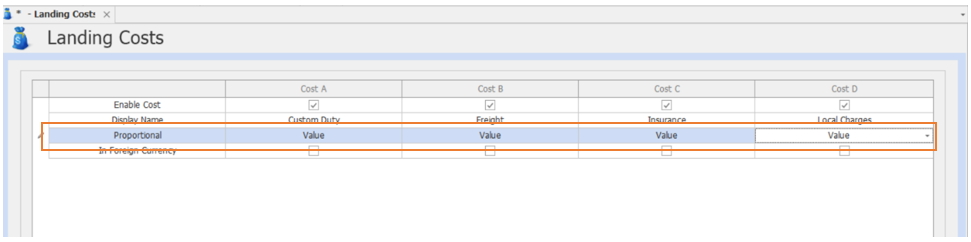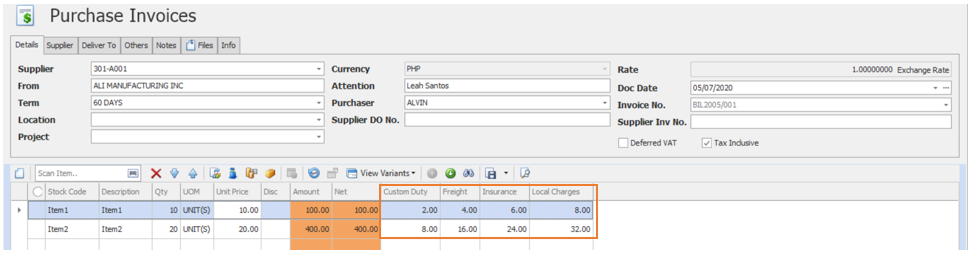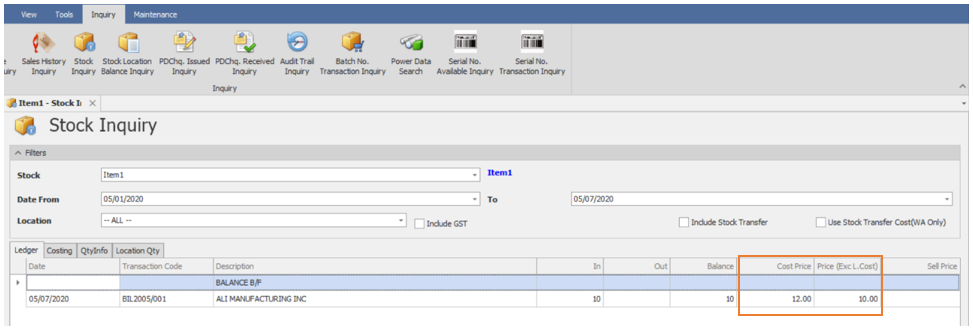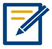# HOW LANDING COST BY VALUE WORKS

Modified on Wed, 15 Jun 2022 at 11:06 PM

HOW LANDING COST BY VALUE WORKS

Overview:

The system is capable of auto-calculating the Landed Cost of an item. There are 4 available ways on how QNE will distribute the Landing Cost and one of it is by using the ‘By Value’ option. ‘By Value’ is computing the landing cost according to its purchased amount. This is the most common option wherein the item that has the highest value will also get the most amount allocation.

Scenario:

Incorporate the Landed Cost in imported stocks and use the purchase value as reference for allocating the total Landing Cost.

Procedure:

Enable ‘Landing Cost’.

1. Go to Purchases > Landing CostsSet the Proportional into ‘Value’.Application:

Issue a Purchase Invoice and use the Landing cost Calculator as shown below. Input the value in the calculator to calculate the amount per Cost.

Set up the Landing Cost by Value in Purchase Invoice.System will automatically compute the Value for Custom Duty, Freight, Insurance, and Local Charges.The calculation of proportion Value is as below:

 Item1 Custom Duty(Subtotal Item1/ Total Amount) x Custom Duty= (PHP 100 /PHP 500) x PHP 10 = 2 php Insurance(Subtotal Item1/ Total Amount) x Insurance= (PHP 100 / PHP 500) x PHP 40= 4 php Freight(Subtotal Item1/ Total Amount) x Freight= (PHP 100 /PHP 500) x PHP 30= 6 php Local Charges (Subtotal Item1/ Total Amount) x Local Charges= (PHP 100 / PHP 500) x PHP 40= 8 php

 Item2 Custom Duty(Subtotal Item1/ Total Amount) x Custom Duty= (PHP 400 /PHP 500) x PHP 10 = PHP 8 Insurance(Subtotal Item1/ Total Amount) x Insurance= (PHP 400 php/ PHP 500) x PHP 20= PHP 16 Freight(Subtotal Item1/ Total Amount) x Freight= (PHP 400 /PHP 500) x PHP 30= 24 php Local Charges (Subtotal Item1/ Total Amount) x Local Charges= (PHP 400 / PHP 500) x PHP 40= 32 php

To check the Cost of the Stock, generate a Stock Inquiry. Under Inquiry> Stock Inquiry.The calculation for the Cost Price is:

Cost Price = (Custom Duty+ Insurance + Freight+ Local Charges)/Qty + Unit Price

= (PHP 2 +PHP 4 +PHP 6 +PHP 8)/10+PHP 10

=PHP 12

 NOTE: